# Types of Triangles

Types of Triangles
Go back to  'Triangles'

Did you know that the triangle is the strongest shape?

It is the most stable and rigid shape used in construction.

Learn about different types of triangle in math such as scalene triangle, isosceles triangle, and equilateral triangle as you scroll down. The chapter also talks about the types of triangles and their properties, types of angles, the perimeter of a triangle, and the area of a triangle.

Let's see some examples of the triangle shape that we see around us.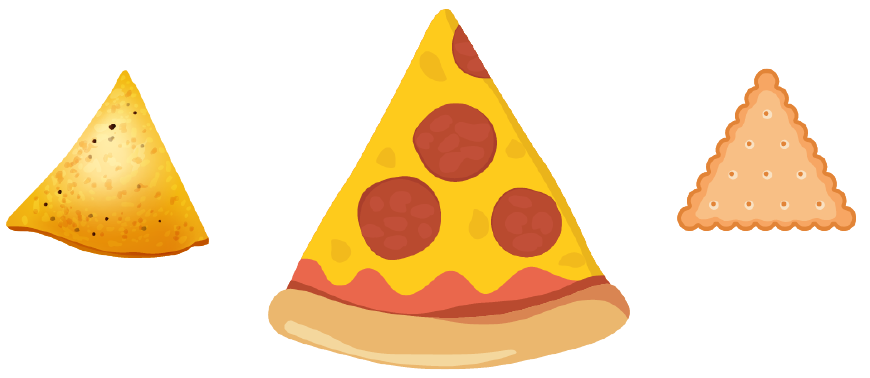A pizza slice and nachos are two of the yummiest triangular-shaped food item we see.

## Lesson Plan

 1 What Is a Triangle? 2 Important Notes 3 Think out of the Box! 4 Solved Examples on Types of Triangle 5 Interactive Questions on Types of Triangle

## Exploring Types of Triangles in Math

Here's an activity for you. You can now visualize various types of triangles in math based on their sides and angles. Try changing the position of the vertices to understand the relationship between sides and angles of a triangle.

## What Is a Triangle?

A triangle is a 2-dimensional geometric shape with three sides and three vertices. We deal with various types of triangles in math, we will study them all as we proceed.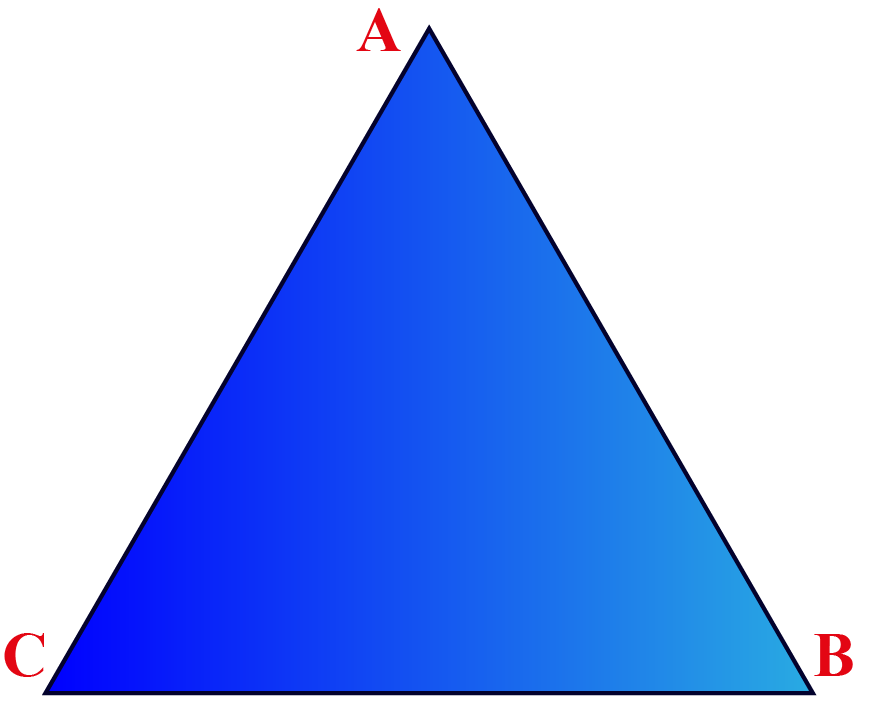The vertices of the given triangle are A, B, and C.

The sides of the given triangle are AB, BC, and AC.

Further, we will study the types of triangle and their properties.Think Tank
1. Can a right triangle also be an equilateral triangle?
2. Can an equilateral triangle be an obtuse angle triangle?

## Types of Triangles and Their Classification

There are different types of triangles in math based on their sides and angles.

### Types of Triangles

The below-given types of triangle chart lists the types of triangles by sides and angles.

 Types of Triangle Based on Sides Types of Triangles Based on Angles Equilateral Triangle Acute-Angled Triangle Acute Triangle) Isosceles Triangle Right-Angled Triangle (Right Triangle) Scalene Triangle Obtuse-Angled Triangle (Obtuse Triangle)

## Properties of a Triangle

Few important properties of triangle are given below:

1. Exterior angle property: $$\angle \text{PRS} = \angle P + \angle Q$$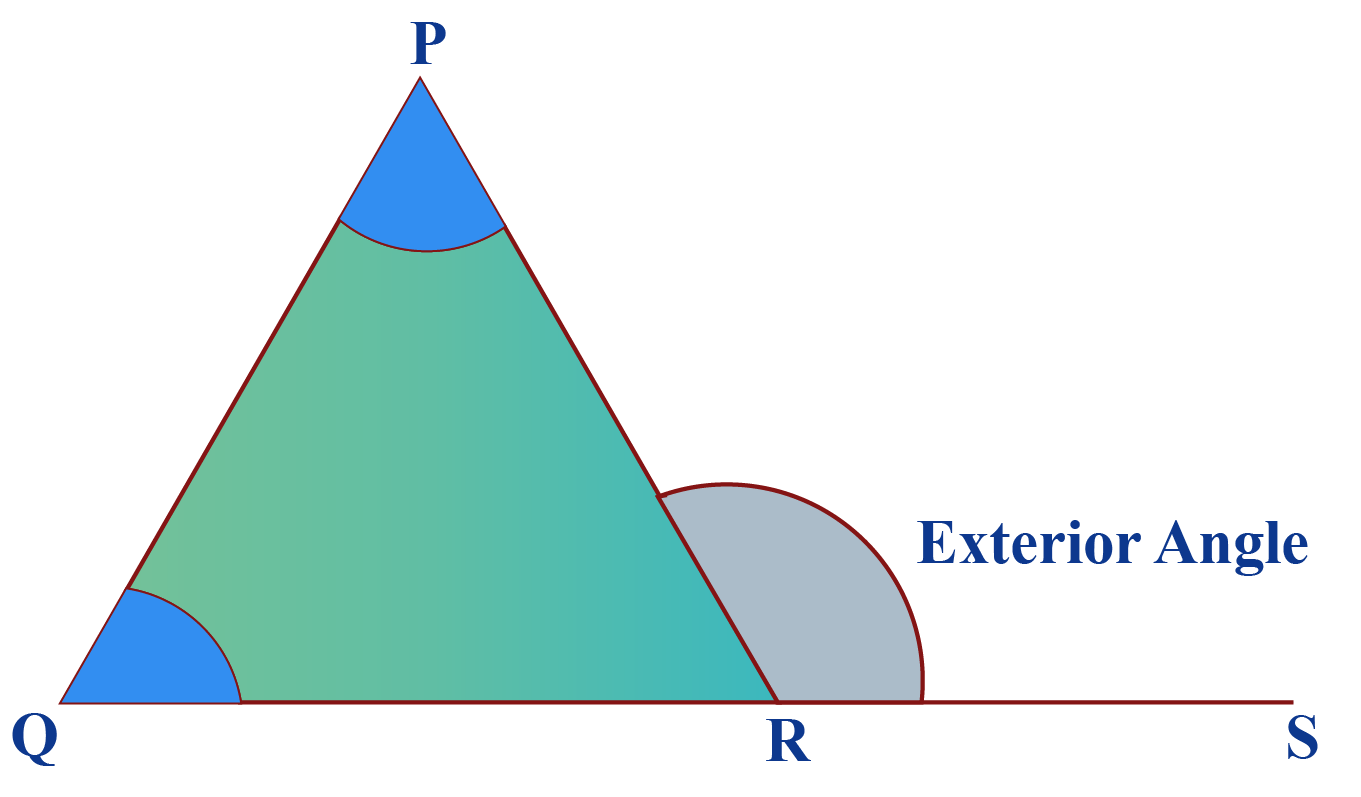2. The area of a triangle is: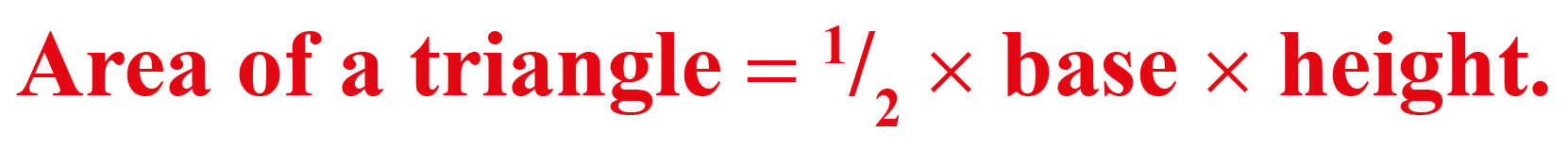3. The perimeter of a triangle is equal to the sum of all the three sides of the triangle. The perimeter of a triangle is directly proportional to the area of a triangle.

## Sides and Angles of a Triangle and Why Are They Important?

Sides and angles are very important aspects of a triangle. We can classify various types of triangles in math by combining sides and angles.

1.There are three types of triangles based on sides. Types of triangles by sides are equilateral triangle, scalene triangle, and isosceles triangle.

2.There are three types of triangles based on angles. Types of triangles by angles are acute-angled triangle, obtuse-angled triangle, and right-angled triangle.

## How to Identify Triangle Type According to the Side Length?

The types of triangle by sides are:

• When all the three sides of a triangle are equal, it is called an equilateral triangle.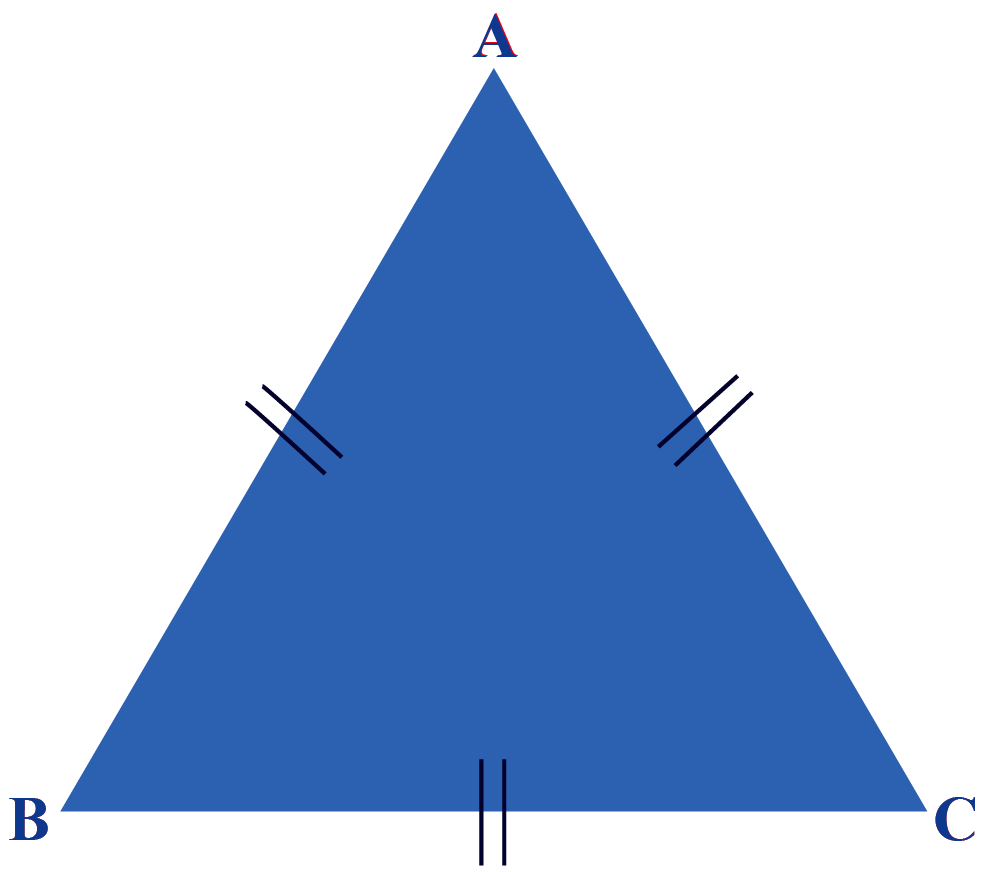The symbol "=" on each side denotes that the sides are equal.

• When two sides of a triangle are equal, it is called an isosceles triangle.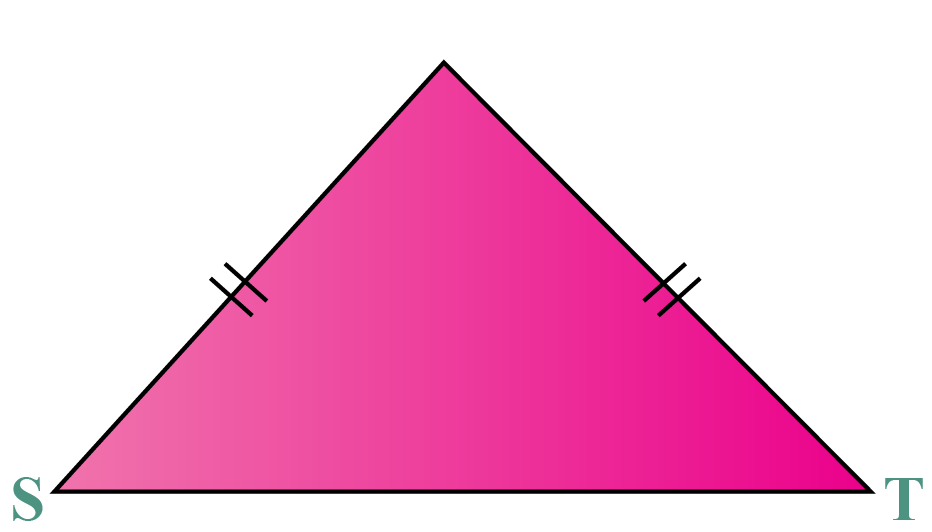In the above triangle, you can see that two sides are marked "=" to denote that those sides are equal.

• When none of the sides of a triangle are equal, it is called a scalene triangle.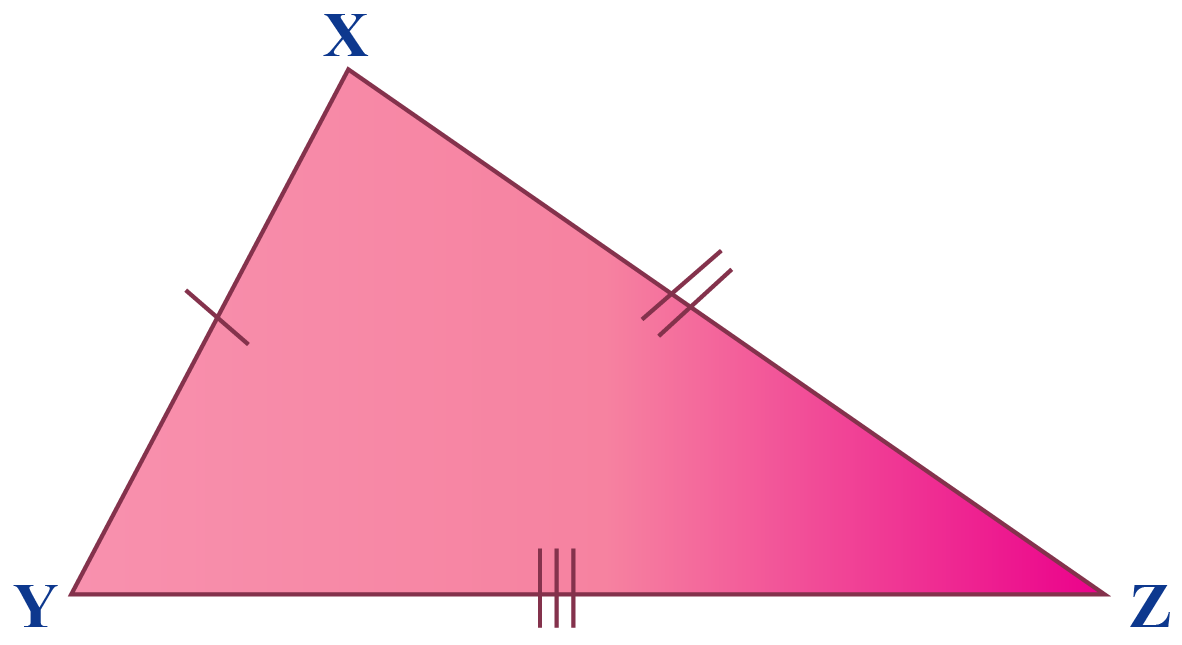In the above triangle, you can observe that all the 3 symbols on the sides are different, which denotes that all the 3 sides are unequal.

## Triangles by Their Angles

• When all the angles of a triangle are acute, it is called an acute-angled triangle or acute triangle.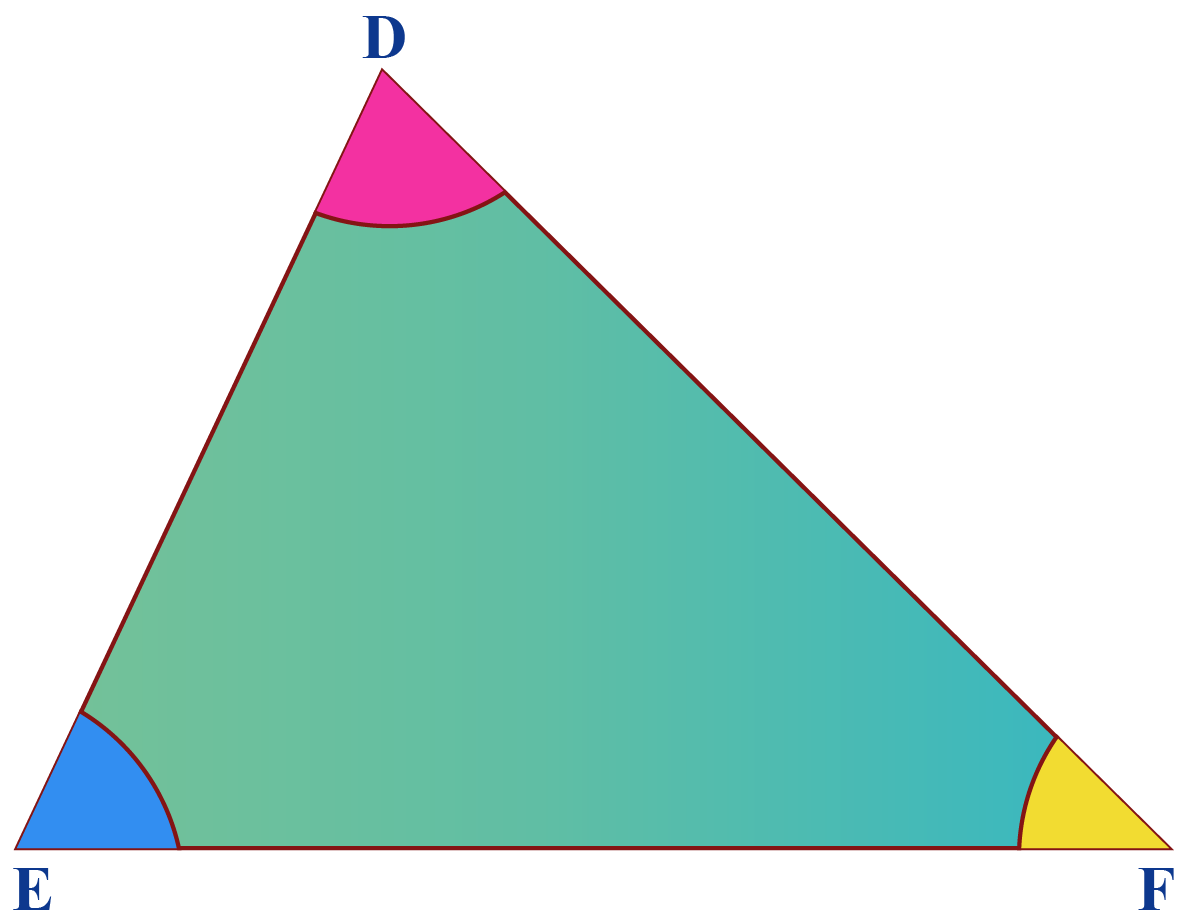• When one of the angles of the triangle is 90°, it is called a right-angled triangle or right triangle.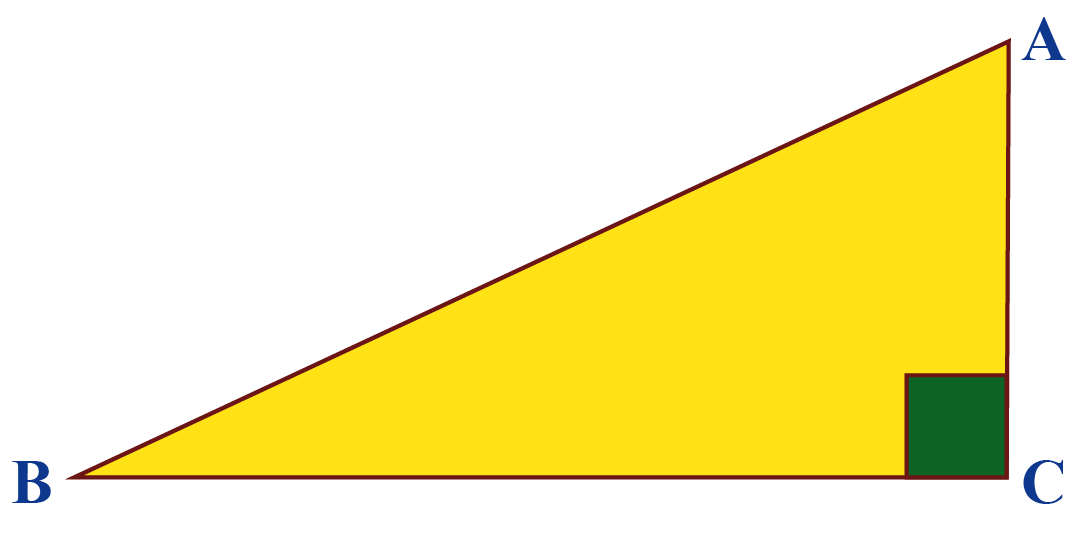In the above image, look at the vertex C. It is marked with a square-shaped symbol.

That denotes a right angle, i.e., 90°

• When one of the angles of a triangle is an obtuse angle, it is called an obtuse-angled triangle or obtuse triangle.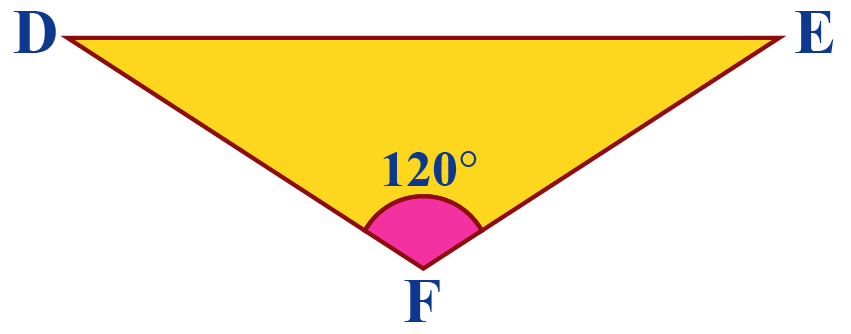## Types of Triangle by Sides and Angles

The combination of all the types of triangles helps us to classify them.

• Equilateral or Equiangular Triangle: When all sides and angles of a triangle are equal, it is called an equilateral or equiangular triangle.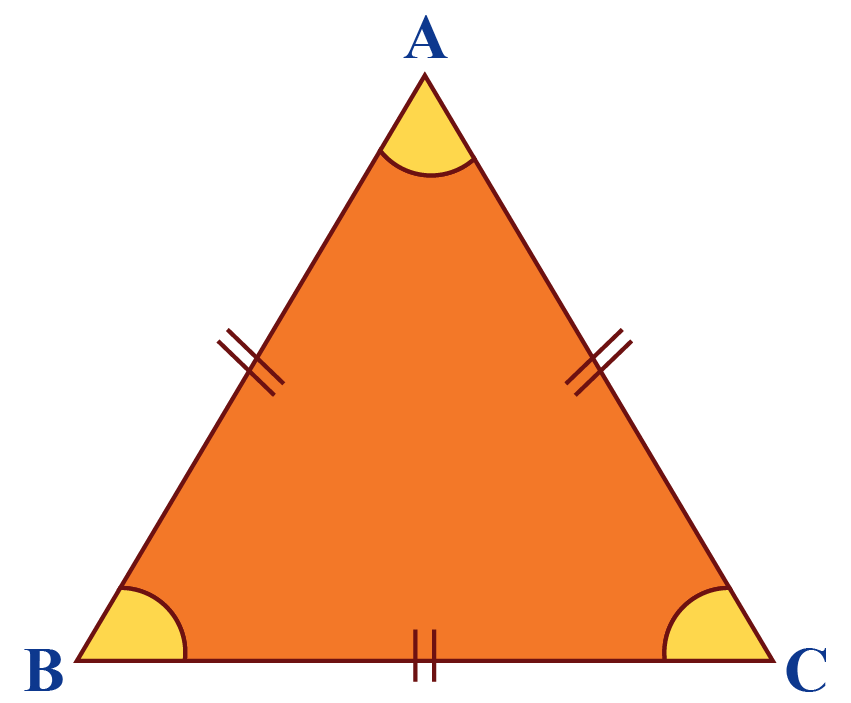• Right Isosceles Triangle: A triangle in which 2 sides are equal and one angle is 900 is called a right isosceles triangle.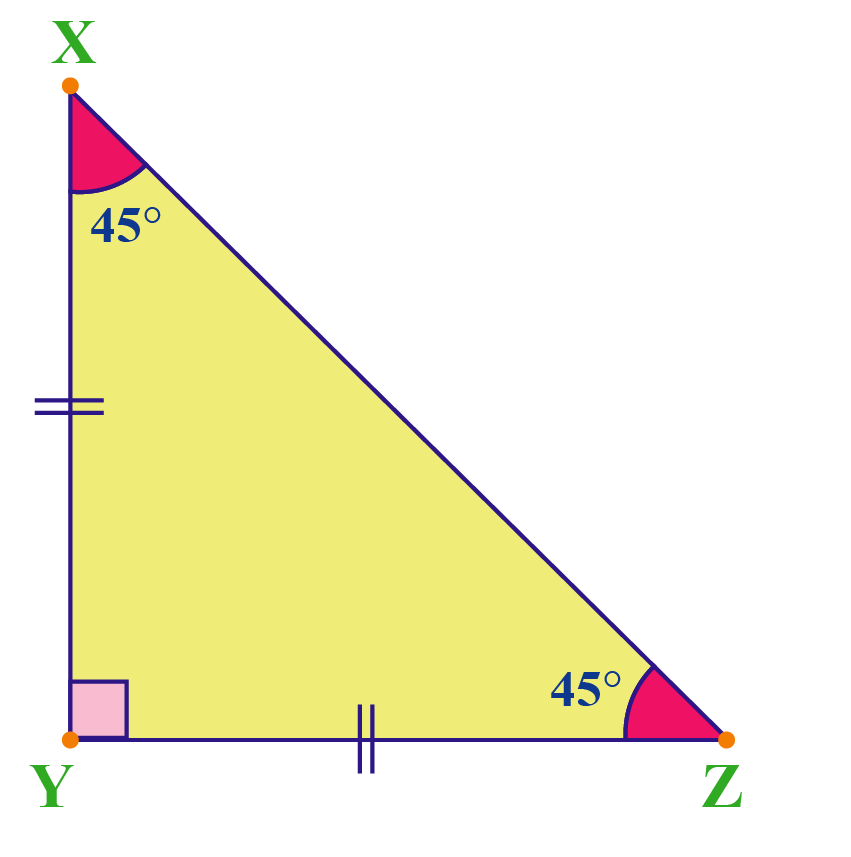• Obtuse Isosceles Triangle: A triangle in which 2 sides are equal and one angle is an obtuse angle is called an obtuse isosceles triangle.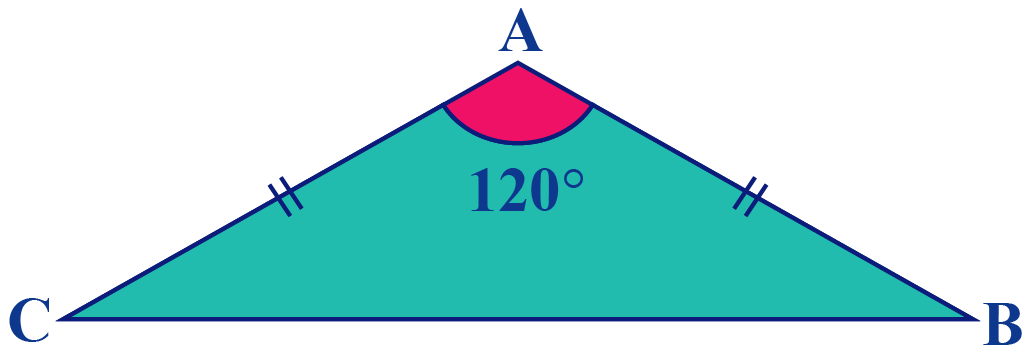• Acute Isosceles Triangle: A triangle in which all 3 angles are acute angles and 2 sides measure the same is called an acute isosceles triangle.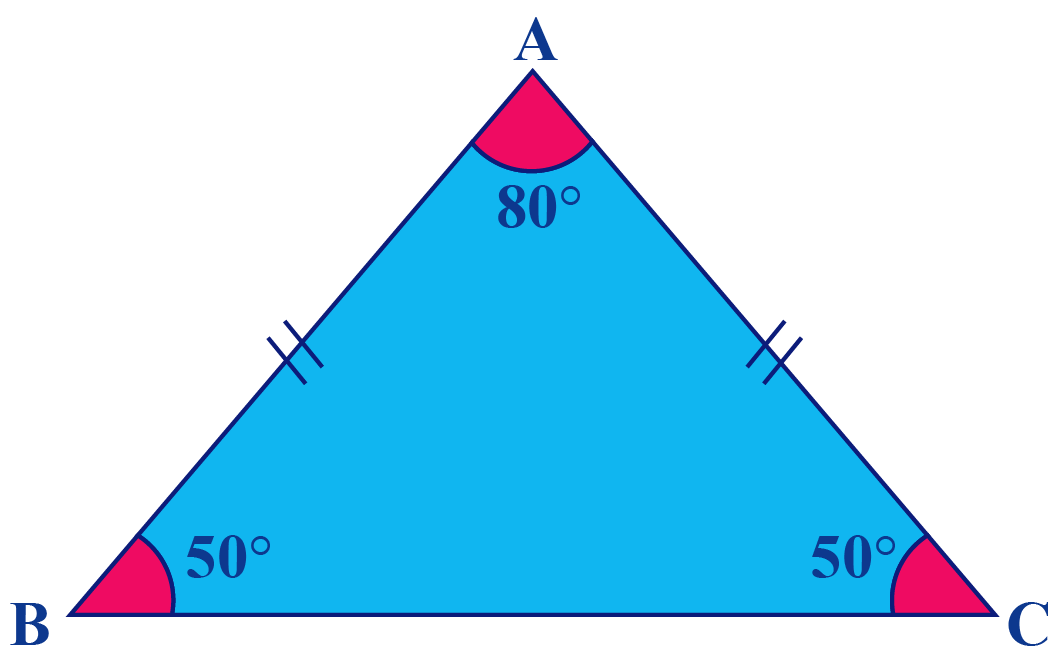• Right Scalene Triangle: A triangle in which all the 3 sides are unequal and any one of the angles is a right angle is called a right scalene triangle.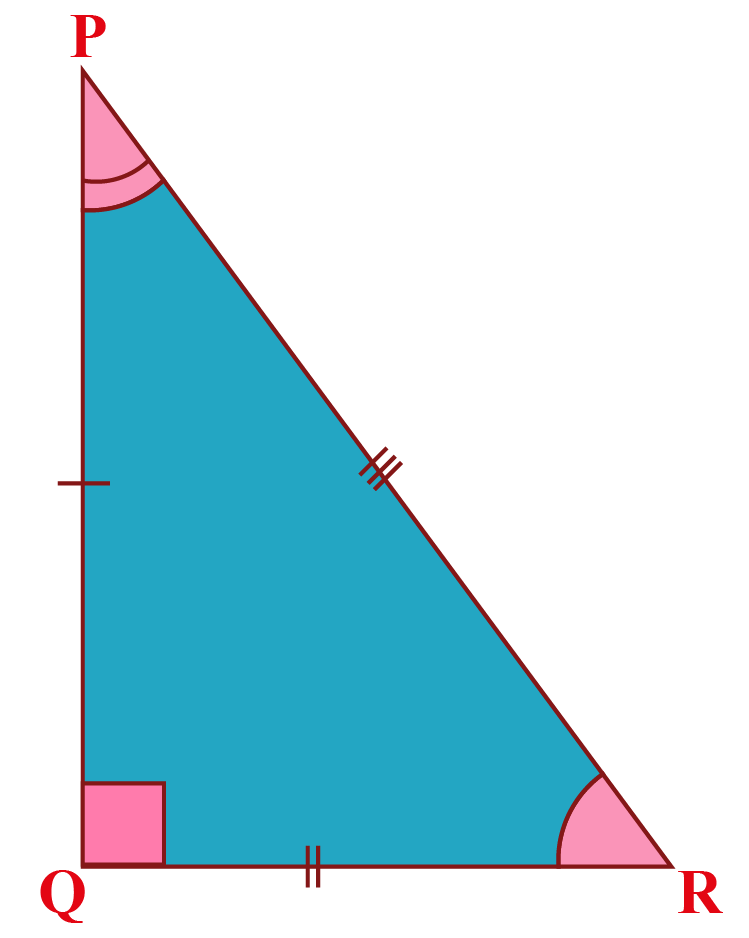• Obtuse Scalene Triangle: A triangle that has one vertex angle as obtuse and all the 3 sides measure different is called an obtuse scalene triangle.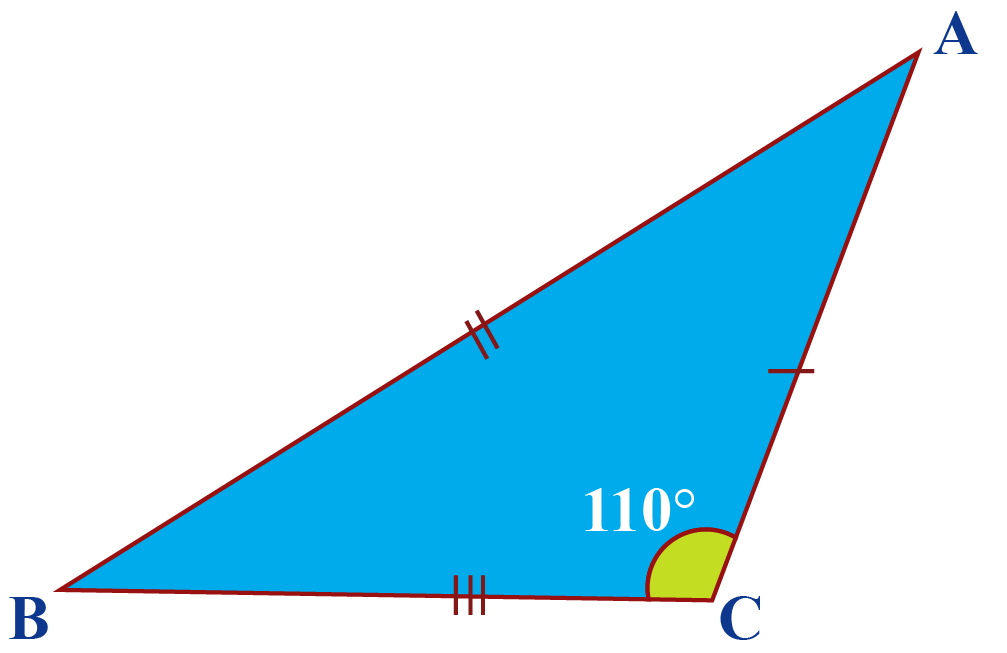• Acute Scalene Triangle: A triangle that has 3 unequal sides and all the angles are acute angles is called an acute scalene triangle.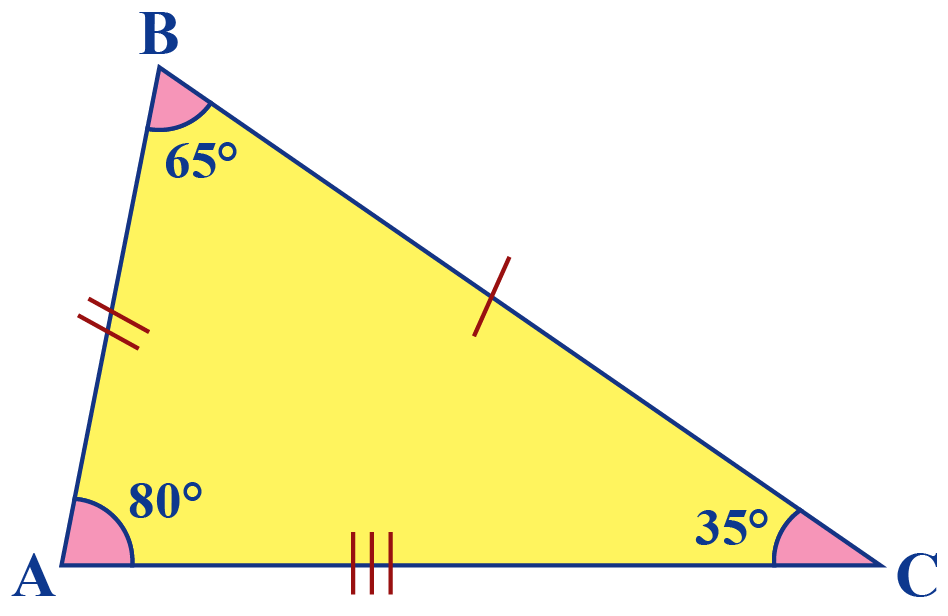Let's now see few problems based on the types of triangles and their properties.

## Solved Examples

 Example 1

What will the measure of each angle in an equilateral triangle?

a. 30°

b. 45°

c. 60°

d. 80°

Solution

All the interior angles are equal in an equilateral triangle.

The sum of the three angles should be 180° in a triangle.

Therefore each side should measure 60°

 $$\therefore$$ Each side should measure 60°.
 Example 2

The length of the two sides of a triangle is equal. Identify the type of triangle.

a. Equilateral triangle

b. Scalene triangle

c. Isosceles triangle

d. Acute angled triangle

Solution

In an equilateral triangle, all the three-length of all the three sides of the triangle will be equal.

In a scalene triangle, the length of none of the sides of the triangle will be equal.

In an isosceles triangle, the length of two sides of the triangle will be equal.

Acute angled triangle is a classification based on angles.

 $$\therefore$$ It is an isosceles triangle.
 Example 3

Alvin gave the measure of vertex angles of a triangle as 81°, 90°, and 70°. Check these measures right or wrong.

Solution

The sum of the angles of the triangle should be 180°

$81^ {\circ}+90^ {\circ}+70^ {\circ}=241$

This is more than $$180^ {\circ}$$

We cannot have a triangle with the vertex angles of $$81^ {\circ}, 90^ {\circ},$$ and $$70^ {\circ}$$.

 $$\therefore$$ The measures given by Alvin are wrong.
 Example 4

Edward drew this triangle in the math class. Identify the type of triangle according to the side lengths given.

a. Equilateral Triangle

b. Scalene Triangle

c. Isosceles Triangle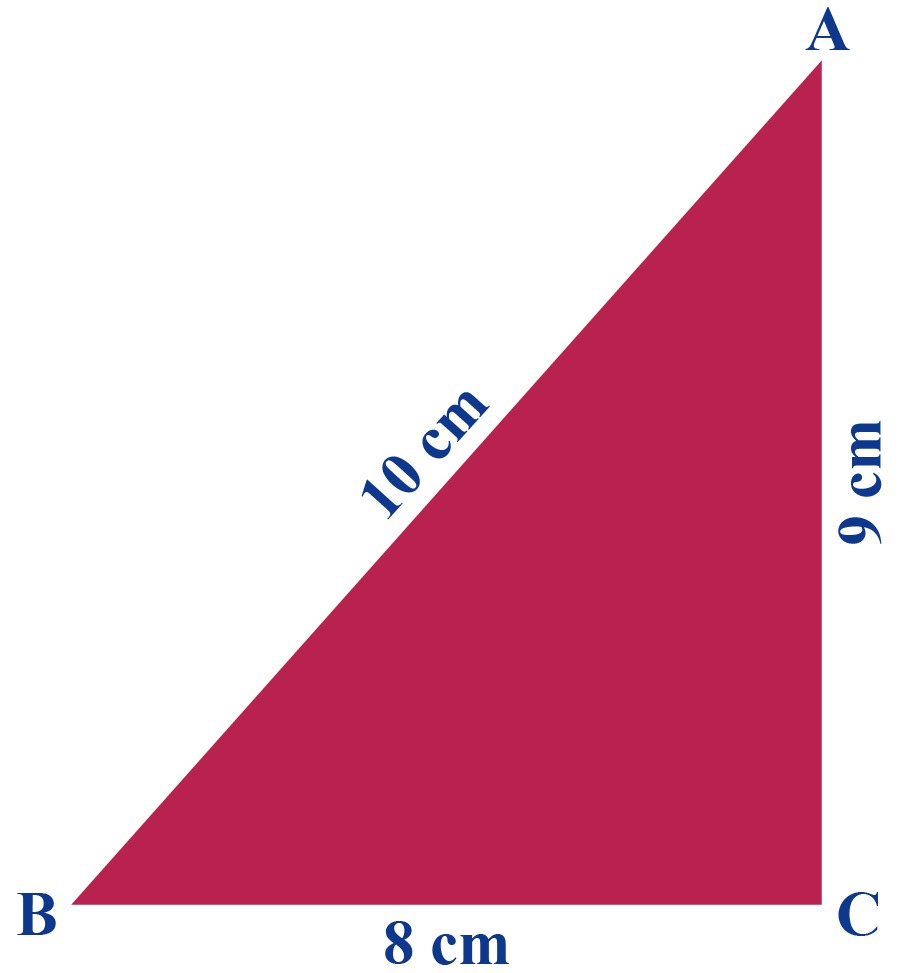Solution

Edward has drawn a triangle giving different lengths for each side.

AB = 10 cm, BC = 8 cm, and CA = 9 cm

 $$\therefore$$ $$\triangle$$ABC is a scalene triangle.
 Example 5

A triangle has 3 equal sides and 3 equal angles. What is the name of the triangle?

a. Equilateral triangle

b. Isosceles triangle

c. Scalene triangle

Solution

In an isosceles triangle, only two sides are equal.

In a scalene triangle, no sides are equal.

In an equilateral triangle, all three sides and angles are equal.

 $$\therefore$$ The correct answer is equilateral triangle.

## Interactive Questions

Here are a few activities for you to practice. Select/Type your answer and click the "Check Answer" button to see the result.

## Let's Summarize

The mini-lesson targeted the fascinating concept of types of triangle in math. The math journey around types of triangle and their properties starts with what a student already knows, and goes on to creatively crafting a fresh concept in the young minds. It is not only relatable and easy to grasp, but will also stay with them forever. Here lies the magic with Cuemath.

At Cuemath, our team of math experts is dedicated to making learning fun for our favorite readers, the students!

Through an interactive and engaging learning-teaching-learning approach, the teachers explore all angles of a topic.

Be it worksheets, online classes, doubt sessions, or any other form of relation, it’s the logical thinking and smart learning approach that we, at Cuemath, believe in.

## 1. Which triangle has two congruent sides?

The angles opposite to the two sides of the same length are congruent.

An isosceles triangle has two congruent sides.

## 2. Name a triangle that has a curved side?

A hyperbolic triangle is a triangle in the hyperbolic plane and it usually has curved sides.

## 3. Does an equilateral triangle have equal angles?

Yes, an equilateral triangle has 3 equal angles.

Each of the three angles is 60 degrees. They add up to 180 degrees.

More Important Topics
Numbers
Algebra
Geometry
Measurement
Money
Data
Trigonometry
Calculus